# 凭借SpringBoot整合Neo4j，我理清了《雷神》中错综复杂的人物关系

xbkong

0收藏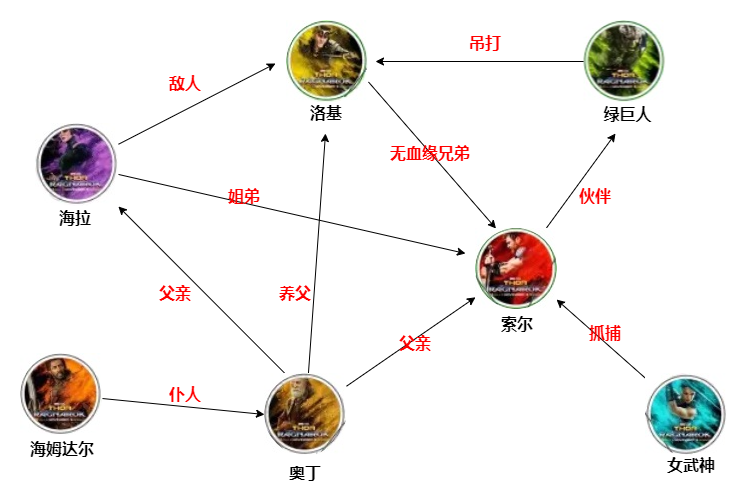• 图数据库neo4j安装
• 简单CQL入门
• springboot整合neo4j
• 文本SPO抽取
• 动态构建知识图谱

### Neo4j安装

``neo4j console``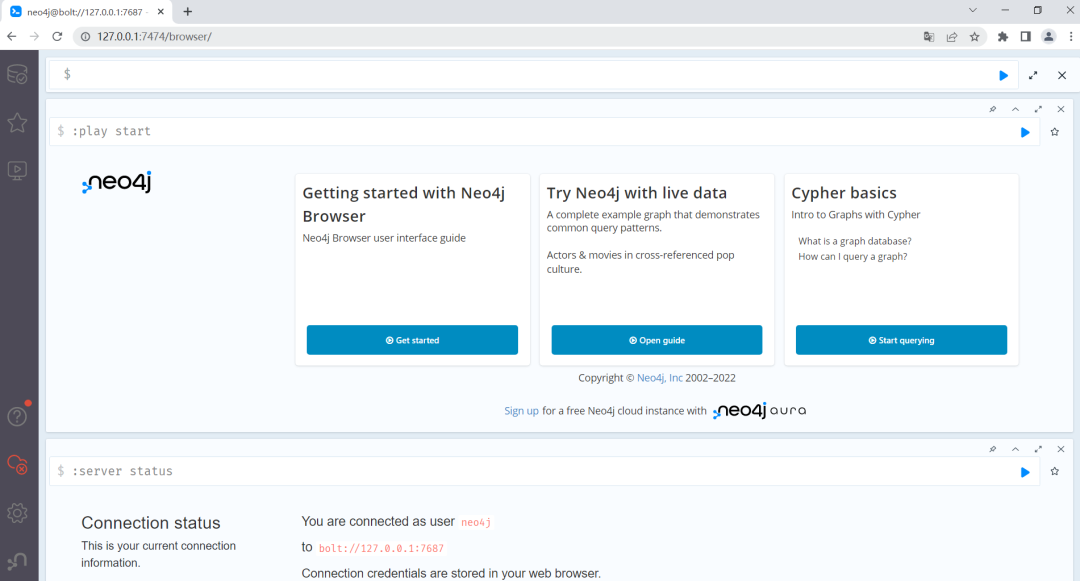### 简单CQL入门

#### 添加节点

``CREATE (<node-name>:<label-name>)``

``CREATE (索尔:Person)``

``````CREATE (
<node-name>:<label-name>
{
<key1>:<value1>,
…
<keyN>:<valueN>
}
)``````

``CREATE (洛基:Person {name:"洛基",title:"诡计之神"})``

#### 查询节点

``MATCH (<node-name>:<label-name>)``

``MATCH (p:Person) RETURN p``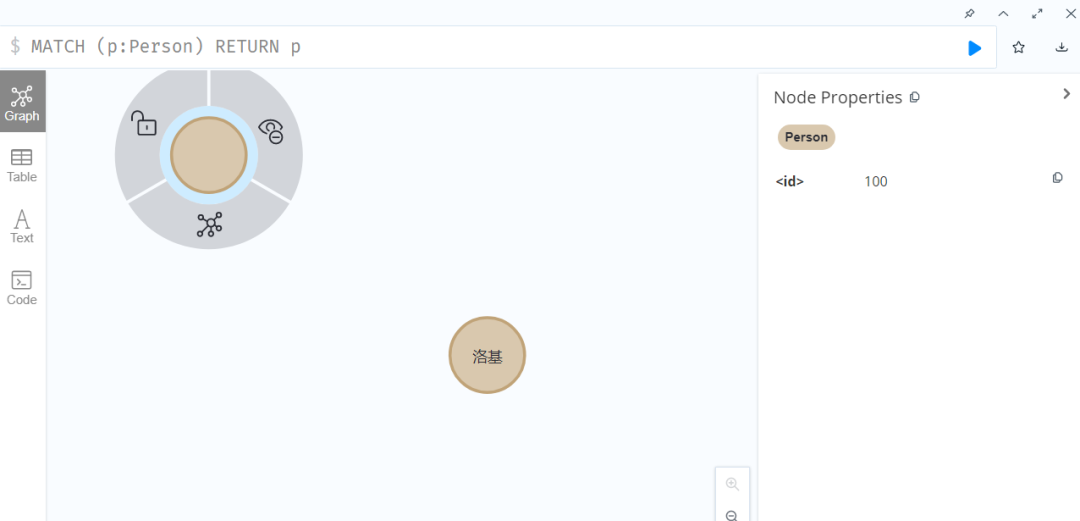#### 删除节点

``````MATCH (p:Person) WHERE id(p)=100
DELETE p``````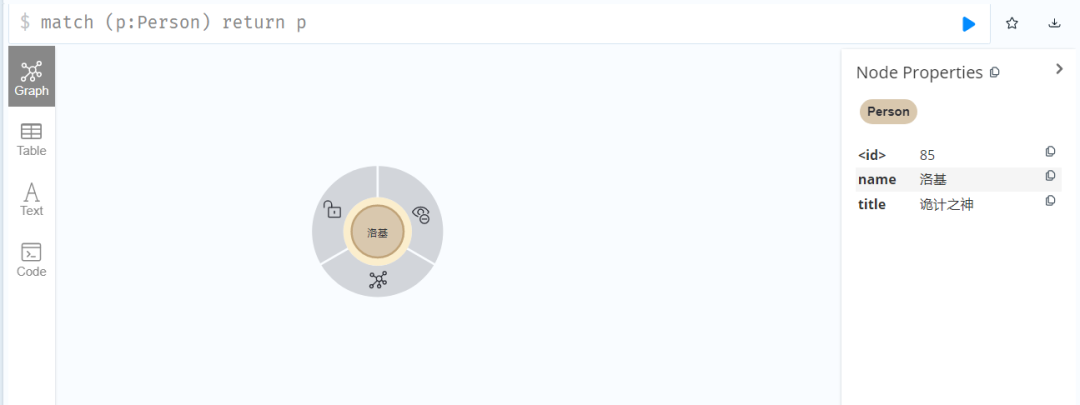#### 添加关联

``CREATE (p:Person {name:"索尔",title:"雷神"})``

``````CREATE (<node-name1>:<label-name1>)
- [<relation-name>:<relation-label-name>]
-> (<node-name2>:<label-name2>)``````

``````MATCH (m:Person),(n:Person)
WHERE m.name='索尔' and n.name='洛基'
CREATE (m)-[r:BROTHER {relation:"无血缘兄弟"}]->(n)
RETURN r``````

``````MATCH (m:Person)-[re:BROTHER]->(n:Person)
RETURN m,re,n``````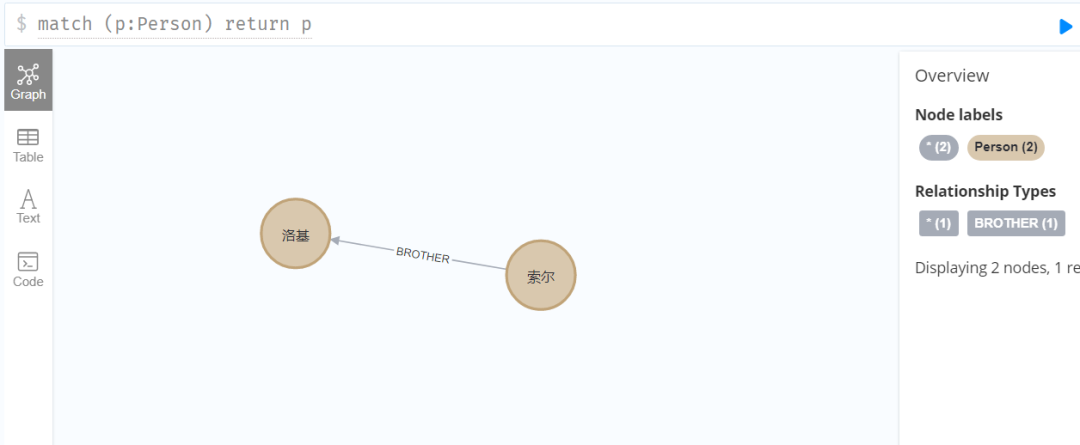``````Neo.ClientError.Schema.ConstraintValidationFailed
Cannot delete node<85>, because it still has relationships. To delete this node, you must first delete its relationships.``````

``````MATCH (m:Person)-[r:BROTHER]->(n:Person)
DELETE m,r``````

### SpringBoot整合Neo4j

``````<dependency>
<groupId>org.springframework.boot</groupId>
<artifactId>spring-boot-starter-data-neo4j</artifactId>
</dependency>``````

``````spring:
data:
neo4j:
uri: bolt://127.0.0.1:7687

#### 节点实体

``````@Data
@NodeEntity(label = "Person")
public class Node {
@Id
@GeneratedValue
private Long id;

@Property(name = "name")
private String name;

@Property(name = "title")
private String title;
}``````

#### Repository持久层

``````@Repository
public interface NodeRepository extends Neo4jRepository<Node,Long> {
@Query("MATCH p=(n:Person) RETURN p")
List<Node> selectAll();

@Query("MATCH(p:Person{name:{name}}) return p")
Node findByName(String name);
}``````

``````@Service
@AllArgsConstructor
public class NodeServiceImpl implements NodeService {
private final NodeRepository nodeRepository;

@Override
public Node save(Node node){
Node save = nodeRepository.save(node);
return save;
}
}``````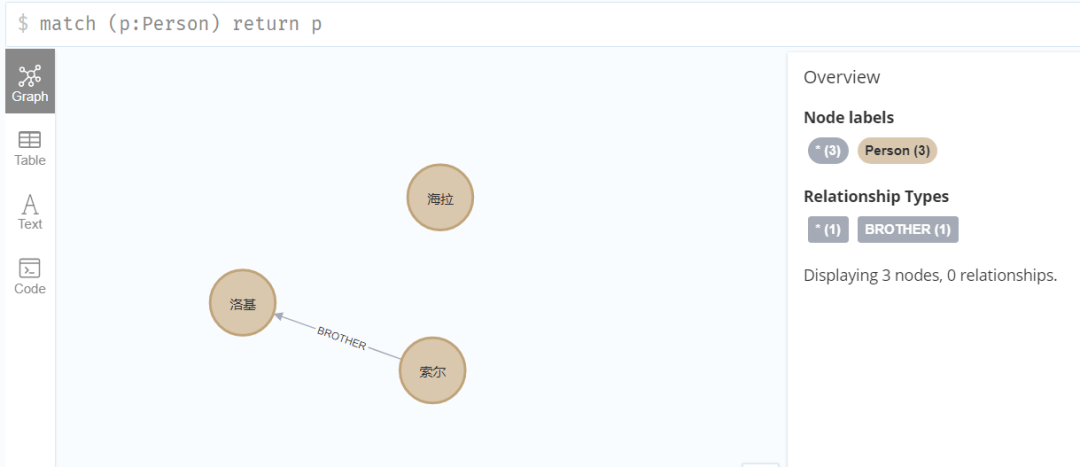``````@Override
public List<Node> getAll(){
List<Node> nodes = nodeRepository.selectAll();
nodes.forEach(System.out::println);
return nodes;
}``````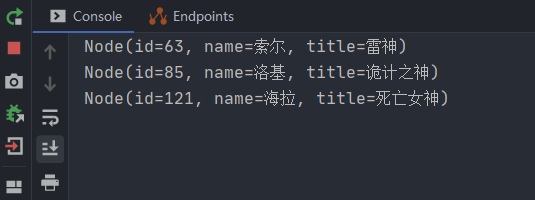#### 关联关系

``````@Data
@RelationshipEntity(type = "Relation")
public class Relation {
@Id
@GeneratedValue
private Long id;

@StartNode
private Node startNode;

@EndNode
private Node endNode;

@Property
private String relation;
}``````

``````@Repository
public interface RelationRepository extends Neo4jRepository<Relation,Long> {
@Query("MATCH p=(n:Person)-[r:Relation]->(m:Person) " +
"WHERE id(n)={startNode} and id(m)={endNode} and r.relation={relation}" +
"RETURN p")
List<Relation> findRelation(@Param("startNode") Node startNode,
@Param("endNode") Node endNode,
@Param("relation");
}``````

#### 创建关联

``````@Override
public void bind(String name1, String name2, String relationName){
Node start = nodeRepository.findByName(name1);
Node end = nodeRepository.findByName(name2);

Relation relation =new Relation();
relation.setStartNode(start);
relation.setEndNode(end);
relation.setRelation(relationName);

relationRepository.save(relation);
}``````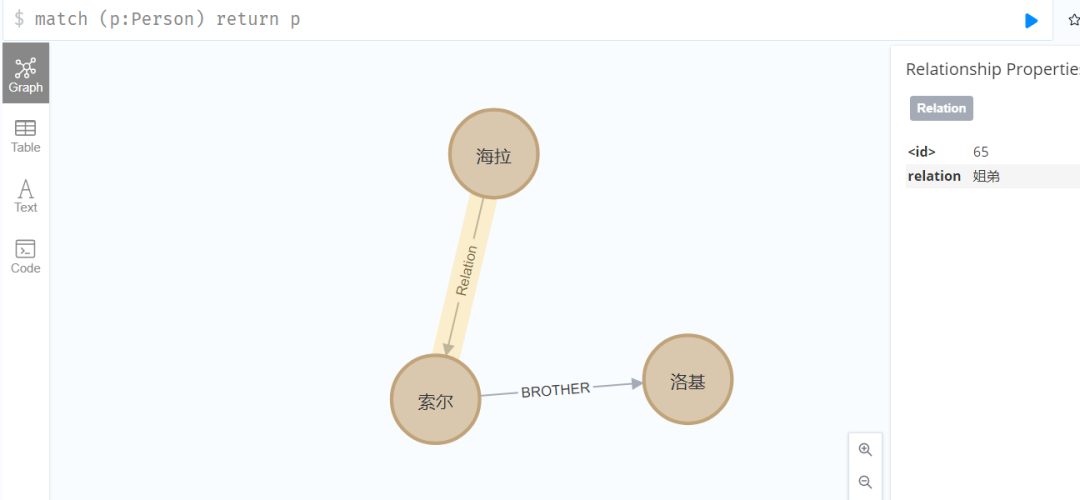### 文本SPO抽取

``````<dependency>
<groupId>com.hankcs</groupId>
<artifactId>hanlp</artifactId>
<version>portable-1.2.4</version>
</dependency>
<dependency>
<groupId>edu.stanford.nlp</groupId>
<artifactId>stanford-parser</artifactId>
<version>3.3.1</version>
</dependency>``````

``````public void mpTest(){
String[] testCaseArray = {
"我一直很喜欢你",
"你被我喜欢",
"美丽又善良的你被卑微的我深深的喜欢着……",
"小米公司主要生产智能手机",
"他送给了我一份礼物",
"这类算法在有限的一段时间内终止",
"如果大海能够带走我的哀愁",
"天青色等烟雨，而我在等你",
"我昨天看见了一个非常可爱的小孩"
};
for (String testCase : testCaseArray) {
MainPart mp = MainPartExtractor.getMainPart(testCase);
System.out.printf("%s   %s   %s \n",
GraphUtil.getNodeValue(mp.getSubject()),
GraphUtil.getNodeValue(mp.getPredicate()),
GraphUtil.getNodeValue(mp.getObject()));
}
}``````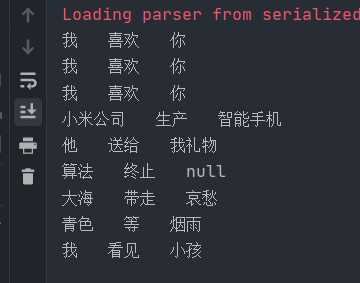### 动态构建知识图谱

``````private Node addNode(TreeGraphNode treeGraphNode){
String nodeName = GraphUtil.getNodeValue(treeGraphNode);

Node existNode = nodeRepository.findByName(nodeName);
if (Objects.nonNull(existNode))
return existNode;

Node node =new Node();
node.setName(nodeName);
return nodeRepository.save(node);
}``````

``````@Override
public List<Relation> parseAndBind(String sentence){
MainPart mp = MainPartExtractor.getMainPart(sentence);

TreeGraphNode subject = mp.getSubject();    //主语
TreeGraphNode predicate = mp.getPredicate();//谓语
TreeGraphNode object = mp.getObject();      //宾语

if (Objects.isNull(subject) || Objects.isNull(object))
return null;

String relationName = GraphUtil.getNodeValue(predicate);//关系词

List<Relation> oldRelation = relationRepository
.findRelation(startNode, endNode,relationName);
if (!oldRelation.isEmpty())
return oldRelation;

Relation botRelation=new Relation();
botRelation.setStartNode(startNode);
botRelation.setEndNode(endNode);
botRelation.setRelation(relationName);
Relation relation = relationRepository.save(botRelation);

return Arrays.asList(relation);
}``````

``````@GetMapping("parse")
public List<Relation> parse(String sentence){
return textAnalysisService.parseAndBind(sentence);
}``````

``````海拉又被称为死亡女神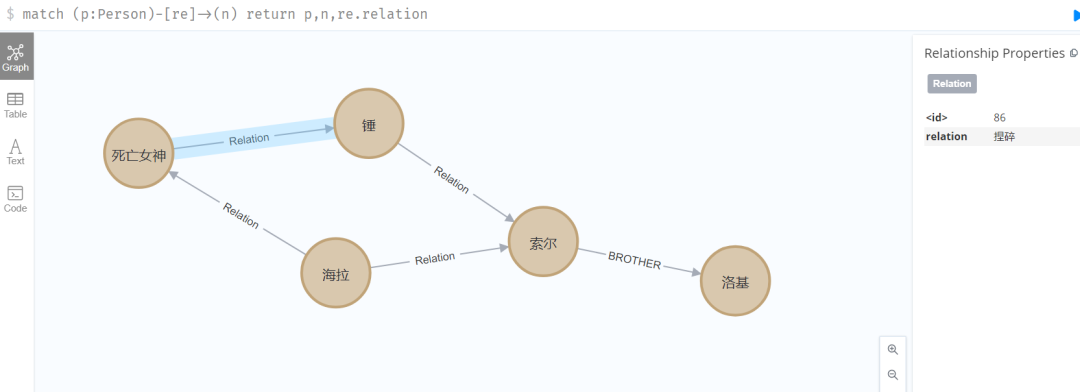• 当前使用的还是单一类型的节点和关联关系，后续可以在代码中丰富更多类型的节点和关联关系实体类
• 文中使用的文本spo抽取效果一般，如果应用于企业项目，那么建议基于更精确的nlp算法去做语义分析
• 当前抽取的节点只包含了实体的名称，不包含具体的属性，后续需要继续完善补充实体的属性
• 完善知识融合，主要是添加实体的指代消解以及属性的融合功能帖子
视频
声望
粉丝
最近发布
社区精华内容